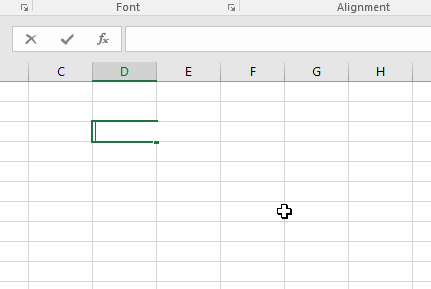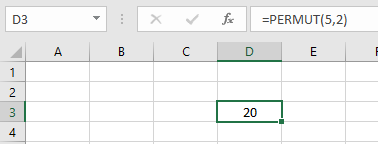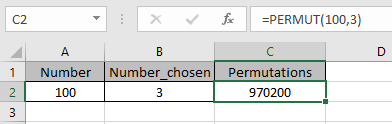# How to use the PERMUT Function in Excel

Permutation is a mathematical operator for getting the number of possible permutations having number (n) & number chosen (r). Order is significant in permutations.
Pnr= FACT(n) / FACT(r)
OR
Cnr= (n)! / (r)!
Factorial function in excel is called FACT function and its mathematical symbol is ! .

The PERMUT function returns the value which is the number of possible permutations. Order is significant in permutations.
Syntax:

=PERMUT (number, number_chosen)

Number : total number of elements or values
Number_chosen : number of values chosen from the total values.
All of this might be confusing so let’s Gear up and understand the PERMUT function using it in an example.

If there are 10 numbers stated 1 to 10. And you get the total number ways selecting any one number from 1 to 10 can be calculated using the PERMUT function.There are 10 ways i.e. 10 possible ways to select one number from the PERMUTation of 10. Order is significant in permutations but here one value is selected so there is no ordered pairing.
Possible values :
1 2 3 4 5 6 7 8 9 10

Now Let’s consider vowels . There are 5 vowels in the alphabet which are a, i, e, o & u.
And we need to find the ordered pair of two vowels

So we need to use the formula to get the Permutations of two vowels.

=PERMUT(5, 2)

5 : five vowels
2 : two vowels PERMUTation requiredThere are 20 possible ways which are
ae ai ao au ea = 5
ei eo eu ia ie = 5
io iu oa oe oi = 5
ou ua ue ui uo = 5
Total = 20
Order of vowels is significant when using the PERMUT function. So ae & ea are counted as different.

Now take a few examples to get the possible permutation having bigger numbers.There are 970200 possible permutations when three different numbers chosen & set in order in a total of 100 numbers.

Note:

• Arguments that contain decimal values are truncated to integers.
• A permutation is a group of values in which order/sequence matters.
• The PERMUT function returns a #VALUE! error value if either argument is not numeric.
• If number is less than number_chosen, the function returns #NUM! error.

As you can get from the above examples that the PERMUT function returns the total number of possible permutations of choosing & then ordering numbers out of total numbers.

Hope you understood how to use PERMUT function in Excel. Explore more articles on Excel COMBIN function
here. Please feel free to state your query or feedback for the above article.

Related Article:

How to use the COMBIN Function in Excel

How to use the FACT Function in Excel

Popular Articles:

50 Excel Shortcuts to Increase Your Productivity

How to use the VLOOKUP Function in Excel

How to use the COUNTIF in Excel 2016

Terms and Conditions of use

The applications/code on this site are distributed as is and without warranties or liability. In no event shall the owner of the copyrights, or the authors of the applications/code be liable for any loss of profit, any problems or any damage resulting from the use or evaluation of the applications/code.